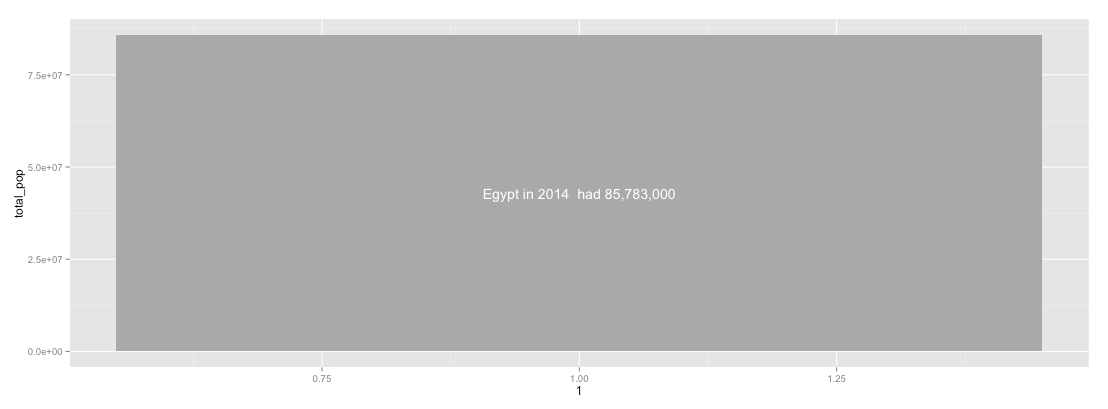# Create Basic Sunburst Graphs with ggplot2

## Solving polar coordinates’ biggest problem of all time (aka text positioning)

You know my love of polar coordinates, right? I do love polar coordinates.

And I love the awesome ggplot2 by the amazing Hadley Wickham (if you are using any other visualization library, you’re missing the point).

In this short tutorial we’ll go through how to create pretty sunburst graphs (at least the basic kind). To get the basic idea, sunburst is a graph that is mainly used to display hierarchical data like a tree map but (you’ve guessed right) it’s circular! I will not talk much about the pros and cons of sunburst graphs, but you can learn a bit more about them here and here

Note that I am not wish to imply that suburst graphs are the best tool to visualize hierarchal data. Because I simply don’t think so .. but that’s another story for another day.

So let’s jump into the action. Let’s assume we have data for the population of Egypt by Governorate (you can find this data here, they removed the data, I had a copy here ). The end result will look like this:

`library(ggplot2) # For awesome vizlibrary(dplyr) # For awesome data wranglinglibrary(scales) # Utilities for scales and formattingpop.eg = read.csv('egypt_pop_2014.csv') # 2 cols: gov,populationsum_total_pop = sum(pop.eg\$population)firstLevel = pop.eg %>% summarize(total_pop=sum(population))sunburst_0 = ggplot(firstLevel) # Just a foundationsunburst_1 =   sunburst_0 +   geom_bar(data=firstLevel, aes(x=1, y=total_pop), fill='darkgrey', stat='identity') +  geom_text(aes(x=1, y=sum_total_pop/2, label=paste('Egypt in 2014  had', comma(total_pop))), color='white')sunburst_1`

This code produces this (dull) bar. Notice here the `aes(x=1)` which is the trick we’ll use to do the multi-levels.Don’t leave now, wait a second.

but when we add

`sunburst_1 + coord_polar(‘y’)`

you get the root of your sunburst. Notice that here `coord_polar` takes an argument `’y’`. Which tells it to rotate around the y axis (not the x axis).

Let’s Add a new layer for governorates

`gov_pop = pop.eg %>% group_by(gov) %>%  summarize(total_pop=sum(population)) %>%  arrange(desc(total_pop))`

The trick we’re going to do here is to use stacked bar chart and then rotate them around the y axis to build to donughts. So let’s do a stacked bar charts

`sunburst_1 + geom_bar(data=gov_pop, aes(x=2, y=total_pop, fill=total_pop), color='white', position='stack', stat='identity', size=0.6) +  geom_text(data=gov_pop, aes(label=paste(gov, total_pop), x=2, y=total_pop), position='stack')`

Notice here the `position=’stack’`, this is the reason why ggplot positioned text properly, without it you will get:

which makes sense if you think about it.

Let’s do a bit of magic

`sunburst_2 + coord_polar('y')`

Not cool, mainly beacuse of text angles and positions. So we need to be slightly smart about it. Mainly we want text to be in the middle if each segment, and to be angled in a readable (not upside down for example).

First let’s fix the text in the middle issue. The position of the text of each governorate should be:

`cumulative_sum - current_value/2`

Let’s compute this and save it in a dataframe

`secondLevel = gov_pop %>%  mutate(running=cumsum(total_pop), pos=running - total_pop/2)`

So sunburst_2 should be

`sunburst_2 = sunburst_1 +  geom_bar(data=secondLevel,    aes(x=2, y=total_pop, fill=total_pop, stroke=3),    color='white', position='stack', stat='identity')sunburst_2_text = sunburst_2 + geom_text(data=secondLevel,   aes(label=paste(gov, comma(total_pop)), x=2, y=pos))`

Better. Let’s go polar again

`sunburst_2_text + coord_polar('y')`

Ok, better. But still the angles are really bad. What to do about that? Let’s compute them!!

We have 4 quadrants, each one .. you know what, code is better than words

`compute_angle = function(perc){  angle = -1  if(perc < 0.25) # 1st q [90,0]    angle = 90 — (perc/0.25) * 90  else if(perc < 0.5) # 2nd q [0, -90]    angle = (perc-0.25) / 0.25 * -90  else if(perc < 0.75) # 3rd q [90, 0]   angle = 90 — ((perc-0.5) / 0.25 * 90)  else if(perc < 1.00) # last q [0, -90]    angle = ((perc -0.75)/0.25) * -90# Or even more compact, but less readable  if(perc < 0.5) # 1st half [90, -90]    angle = (180 - (perc/0.5) * 180) - 90  else # 2nd half [90, -90]    angle = (90 - ((perc - 0.5)/0.5) * 180)  return(angle)}`

Here perc is a variable ranging from 0 to 1 representing the position of the “text label” in a circle.

let’s compute and save agian.

`secondLevel = gov_pop %>%  mutate(running=cumsum(total_pop), pos=running — total_pop/2) %>% group_by(1:n()) %>% # to compute row by row  mutate(angle=compute_angle((running — total_pop/2) / sum_total_pop))`

Let’s do viz again

`sunburst_2_text = sunburst_2 +  geom_text(data=secondLevel,     aes(label=paste(gov, comma(total_pop)),    x=2, y=pos, angle=angle))sunburst_3 + coord_polar('y')`

This would look like

Notice how the text flips on the 6 o’clock to sustain readability. Pretty cool, right? Let’s change colors a bit.

`sunburst_2_text + scale_y_continuous(labels=comma) + scale_fill_continuous(low='white', high='darkred') + coord_polar('y') + theme_minimal()`

Of course there are many problems in the graph. For example, the crowded text for the small governorates, and the overall viz needs to be augmented with a measure of area to tell a compelling story and many more other problems …

… but text angle and positioning are not any of them.

I am still exploring the concept of sunbursts. Adding a new level is obvious, but I still need to explore how to draw a level with missing elements in it (to visualize depth in the hierarchy) like

## I am working on a small project to visualize demographics, inequalities, density and other phenomena of the Egyptian population. If you are interested, shoot me a message.

You can find the code used for this post on gist.github here.

We are a team of data scientists and software engineers working to help enterprises makes the most out of their data. Our projects range from data analysis to extract insights, to predictive analytics to support decision making, to scalable production ready data products. Our focus areas are (1) personalization and (2) operational efficiency.

--

--

## More from Optima . Blog

Thoughts on data, technology, startups, and oftentimes, other things.